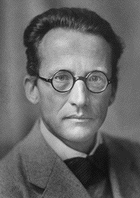# Spectroscopical Term Schemata

 * Structures in Micro Objects *

Term Schema of Electron HullsThe term schema of the electron hull in an arbitrary atom
is obtained by solving the Schrödinger equation
for a sufficiently detailed Coulomb potential.
For any electron state, we denote by
n ... the principal quantum number (shells K,L,... with n = 1,2,...),
l ... the orbital angular momentum (orbitals s,p,... with l = 0,...,n),
m ... the magnetic quantum number (m = -l,...,l).

At Z = 121 the Term Schema becomes irregular,
since not only the 8s-orbital but even a part of the 8p-orbital, 8p(1/2)2,
is located below the 5g- (a totally new feature), 6f-, and 7d-orbitals.
The other part of the 8p-orbital, 8p(3/2)4, however, lies above the 9s- and the lower 9p(1/2)-orbitals.
This is the reason why the 8th and 9th period do not show the famous
Seaborg parallel alignment configuration
which was characteristic for the 6th (lanthanides) and 7th (actinides) period.

# of statesK (n = 1)L (n = 2)M (n = 3)N (n = 4)O (n = 5)P (n = 6)Q (n = 7)R (n = 8)S (n = 9)Elements
172___8p(3/2)4Bi''..Rn''
168___9p(1/2)2Tl'',Pb''
166___9s2Fr'',Ra''
164___7d10Lr'...Hg''
154___6f14Ac'...No'
140___5g18Ubt...Uqn
122___8p(1/2)2Ubu,Ubb
120___8s2Fr',Ra'
118___7p6Uut...Uuo
112___6d10Lr...Uub
102___5f14Ac...No
88___7s2Fr,Ra
86___6p6Tl...Rn
80___5d10Lu...Hg
70___4f14La...Yb
56___6s2Cs,Ba
54___5p6In...Xe
48___4d10Y...Cd
38___5s2Rb,Sr
36___4p6Ga...Kr
30___3d10Sc...Zn
20___4s2K,Ca
18___3p6Al...Ar
12___3s2Na,Mg
10___2p6B...Ne
4___2s2Li,Be
2___1s2H,He

Boldface state numbers denote closed hull shells
and are called magic shell numbers.

 <| Navigation Center <| <| Back to Algebra<| <| Back to Curriculum<|